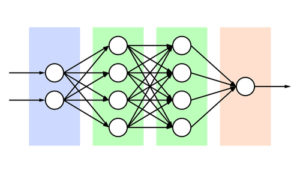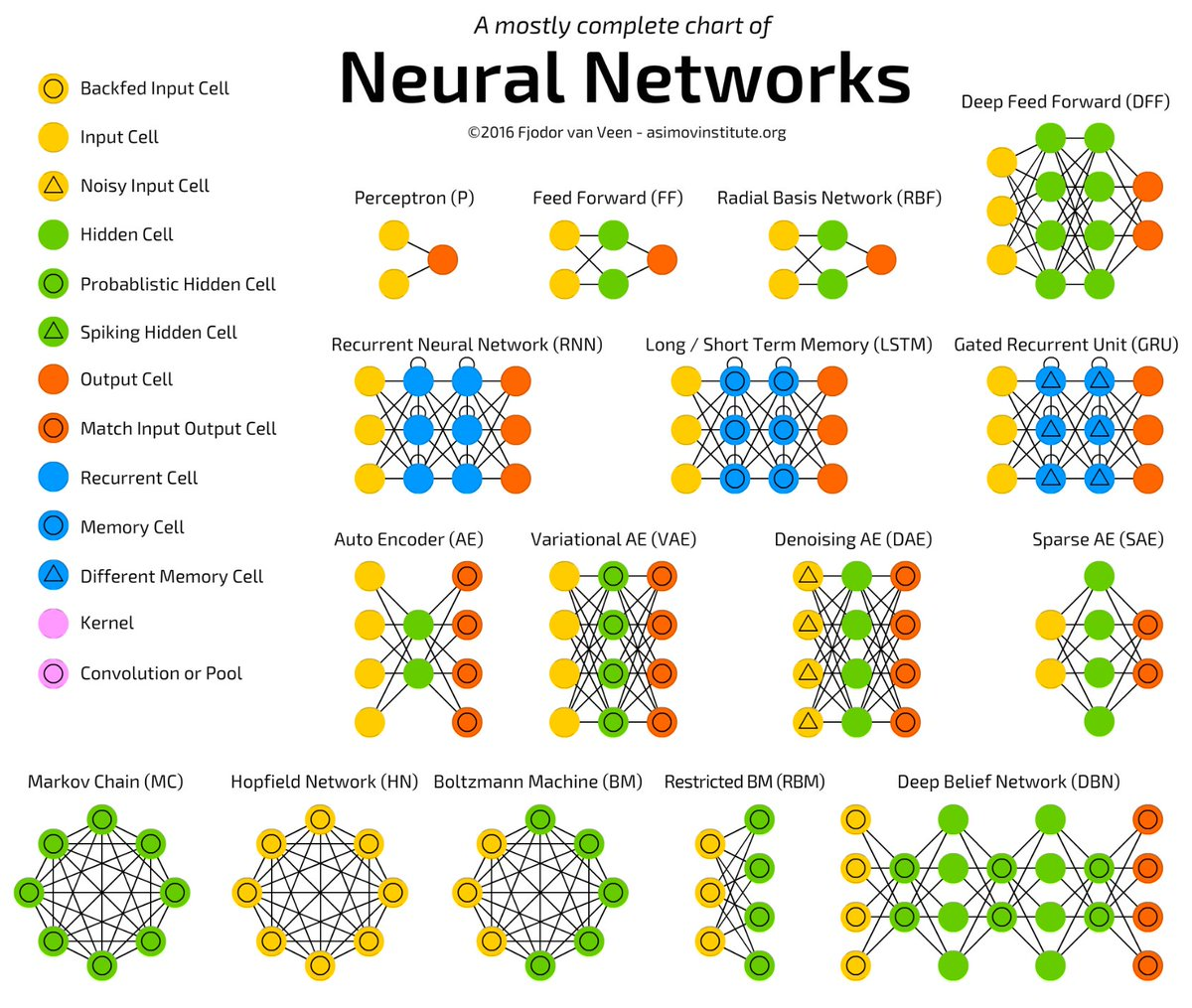## Getting Deeper with Deep Learning: Part 2

In this third part of my Deep Learning series, we will present six different types of neural networks, provide a brief overview of each, then delve a little deeper into each network and its subtypes. Neural networks use mathematical functions found in the calculus and linear algebra realm of mathematics, however, I will try to avoid getting into the math. There are better places to learn this level of mathematics than inside a technical blog. So let’s see how much further down the rabbit hole we can go.

Six basic types of Artificial Neural Networks:

1. Feedforward Neural Network

2. Radial Basis Function Neural Network

3. Kohonen Self-Organizing Neural Network

4. Recurrent Neural Network

5. Modular Neural Network

6. Physical Neural NetworkOne example of a Feedforward Neural network

The Feedforward Neural Network was the first and simplest of all neural networks. In a feedforward neural network, the information moves only from the input layer through the hidden layers to the output layer without any cycles or loops. Each neuron is connected to every neuron in the next layer and no neuron has a connection with other neurons in its own layer. Frequently, the feedforward type will use sigmoidal activation (referring to the sigmoid function in mathematics where the graph is S-shaped) in conjunction with continuous neurons in the context of backpropagation.

Backpropagation:

Backpropagation is a method used to calculate the error contribution of each neuron after a batch of data has been processed, and adjust the weighting of each neuron to complete the learning process for that data. This is because the error is calculated at the output of the neural network and distributed back through the layers (a form of gradient descent). Backpropagation requires a known, desired output for each input value and is therefore considered to be a supervised learning method, although it is used in some unsupervised networks.

The Radial Basis Function Network uses radial basis functions that have a distance criterion with respect to a center. Radial basis functions replace the sigmoidal function in multi-layer neural nets. RBF networks have the disadvantage of requiring good coverage of the inputs with radial basis functions. The centers are determined in reference to the input data, but without reference to the prediction task. As a result, areas of the input space that are irrelevant to the prediction task can waste representational resources. One common solution to this disadvantage is to associate each data point with its own center, however, this can cause an expansion of the linear system to be solved in the final layer and could require shrinkage techniques to avoid overfitting. An overfitted model could decrease the predictive performance of the neural network as it overreacts to minor fluctuations in the training data.

The Kohonen Self-Organizing Neural Network (pronounced Kuh-hoe-nen) was invented by Teuvo Kohonen. This self-organizing neural network is ideal for the visualization of low-dimensional views of high-dimensional data. The network applies competitive learning to a set of input data instead of the error-correction learning utilized by other neural networks. It also has a matrix of neurons that are stimulated by input signals. Those signals should describe some attributes of effects which occur in the surrounding neurons, which should enable the structure of this network to group those effects. The network assigns the neuron with the best match as the winner. At the beginning of the training, the weights are small random numbers and while learning, those weights are adjusted in a way to create an internal structure of input data, however, there is a risk that the neurons could link with some values before groups are correctly recognized, so the learning process should be repeated with different weights. It is necessary that the net can adjust the weights of the winning neuron and its neighbors based on response strength. Network topology is defined by determining the neighbors of each neuron. This type of network can be used in unsupervised learning to find its own solution, however, other programs or users must figure out how to interpret the output.

Unlike the feedforward neural network, the Recurrent Neural Network allows for bi-directional flow of data. A fully recurrent network creates a directed connection between every pair of neurons with each having a time-varying, real-valued (more than just one or zero) activation (output).This network allows for dynamic temporal behavior as training sequences of real-valued input vectors become sequences of activations of the input nodes—one input vector at a time. At every time step, each non-input unit computes its current activation as a nonlinear function of the weighted sum of the activations of all units from which it receives connections. This system can explicitly activate some output units at certain time steps independently of incoming signals. Gradient descent can be used in this model to change each weight in proportion to its derivative with respect to the error, provided the non-linear activation functions are differentiable. The standard method is called “backpropagation through time” or BPTT, a generalization of back-propagation for feedforward networks.

The chart below shows several types of neural networks discussed in this article as well as several not discussed.The Modular Neural Network is a particularly interesting type of neural network as it consists of multiple independent neural networks that are moderated by an intermediary network. A modular network can also work in different ways. One type of modular neural network can work on separate subtasks of the overall main task with the intermediary processing the subtask results to create the final output. Another way that a modular neural network can work is that each independent network can process the same inputs and then ‘vote’ on the correct output for the task. This method usually involves the weights on the neurons being different from one independent network to the next, and then comparing to see if the networks still arrive at the same results.

The Physical Neural Network utilizes physical hardware connected by electrically adjustable resistance material to emulate the function of the synapse. An example of this is the ADALINE memristor-based neural network developed by Stanford professor Professor Bernard Widrow and his graduate student Ted Hoff, in 1960. ADALINE was based on the McCulloch-Pitts neuron (the standard) and consisted of a weight, a bias, and a summation function. However, the difference between ADALINE and the standard neuron is that in the learning phase, the weights are adjusted according to the weighted sum of the inputs (the net). In the standard neuron, the weighted sum is passed to the activation function and the function’s output is used to adjust the weights.

I hope this information has provided a better understanding of the subject, but obviously, there is much more to be mined from the study of these various neural networks. We encourage you to seek out other reliable sources that will also enhance your Deep Learning understanding.

Previous post in the Deep Learning series: Getting Deeper with Deep Learning: Part 1